Shell ScriptingGenerate Random Passwords in Shell Scripting via Character Array Method

# Generate Random Passwords in Shell Scripting via Character Array Method

In the previous article, we have learned how to generate random passwords using two methods:

1. The mkpasswd command.
2. Calculating the Hash for a random data (or number), then cutting characters (with length matching the required password length) from the resulting hash.

Now, consider the case wherein the Linux (or UNIX) distribution running on your server doesn’t support either the mkpasswd command, or the Hash computing tool. What will you do in this case?!

– Mmm, well!! I will search google for this problem, trying to find some other tool, or at the end I may create the passwords myself.

Okay, I am with you in the first, but the second seems non-realistic!!!

Using the Characters Array Method

The idea is simple:

• First: we are going to create an array, and initialize it with all the characters in the possible character-space (that we may want to see in our generated passwords), one character per array element.
• Second: we will generate a random integer in the range between 0 and the array length minus 1.
• Third: the random number generated from the previous step will be used as an index to the array. The character stored in the array element at this index will be read, and added to the required password.
• Fourth: the previous two steps (Second and Third) will be repeated a number of times equal to the length of the required password.
• Fifth: Congratulations!! You have now a password consisting of randomly chosen characters.

This is the logic of the method. Now, we need to implement it in code!!

First: to create the characters array, I am going to create an array for each type of characters: one for digits, another for the lowercase letters, then a third for the uppercase letters.

```digits=({2..9})
lower=({a..k} {m..n} {p..z})
upper=({A..N} {P..Z})```

Next, I will concatenate them.

`CharArray=(\${digits[*]} \${lower[*]} \${upper[*]})`

Now, we have an array consisting of all the characters in the desired character-space for the random passwords we need to generate. Let’s print the entire array to make sure we are going in the right direction: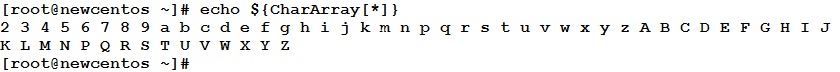Nice!! Now we sh…

– What is the Nice?! There are missing character!! where are 0 and 1?! Where are the small and capital O?!

Ah, there is also one that you forgot to ask about: the l (small L) letter. The reason is clear: could you differentiate between 1 and l?! I bet you couldn’t!!! The former 1 is one, while the latter is (small L). And there will be a similar issue between 0 (zero) and the small and capital O.

Could you imagine what this confusion may do when the matter is related to passwords?! Notice that any typo will not be tolerated. It is a password!! If we were in a normal text typing, it wouldn’t be a problem at all; the wrong character will be identified and understood easily from the whole context of the speech. But, unfortunately this is not the case (and it shouldn’t be).Second: A random number is to be generated between 0 and the index of the last element in the array. This index is equal to array length minus 1. So, our intermediate goal is to get the length of the characters array.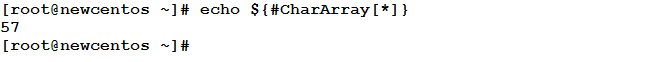To generate the required random number in the range between 0 and 57 (excluding the upper limit 57), we could use the formula we learned in the Generating Random Numbers article:

`R=\$((\$RANDOM%Y))`

Where Y in our case will be equal to 57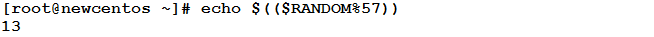Okay. Now, we have in hand a random number in the desired range. What is next?!

Third: the Array element at index 13 will be read.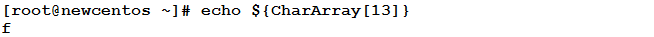This is the first character in the required password.

Fourth: based on the length of the required password, the Second and Third steps should be repeated until we get the desired number of characters. The best way for that is using a for loop.

Example
Let’s write the previous procedure in a script.

```#!/bin/bash
#This script optionally accepts a password length from the user
#as a command-line argument, and creates a random password with
#that length.
#Author: Eduonix
#Date: October 2015
if [ \$# -eq 1 ]; then
length=\$1
else
length=8
fi
digits=({2..9})
lower=({a..k} {m..n} {p..z})
upper=({A..N} {P..Z})
CharArray=(\${digits[*]} \${lower[*]} \${upper[*]})
ArrayLength=\${#CharArray[*]}
for i in `seq 1 \$length`
do
index=\$((\$RANDOM%\$ArrayLength))
char=\${CharArray[\$index]}
done

Let’s see it in action: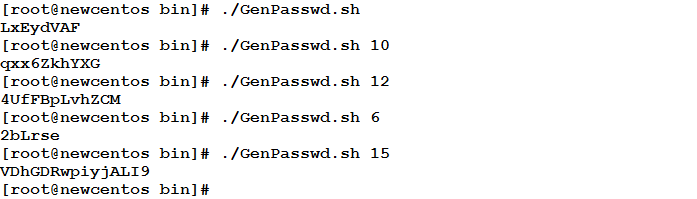Perfect!!!

Summary
In this article, we have learned the third method to generate random passwords: the Character array method.
See you in the next article.

#### 1 COMMENT

1.Joshua Mertens

In a simple but concrete way, explained the importance of owning a good password. Article abounds with concrete examples. Very professionally done.

### Why Is Design Thinking Important In Digital Transformation?

21,501Fans
4,210Followers
104,000Subscribers

### Why Is Design Thinking Important In Digital Transformation?

- Advertisement -